# Bar Graph – Examples, PDF

Statistics is a very complicated and complex tool that requires the person to work out logical connections and codes from the gathered and collected data. People have created various methods and ways that will make this process efficient and less time-consuming, one of which is called the bar graph.

## 1. Bar Graph and Pictographs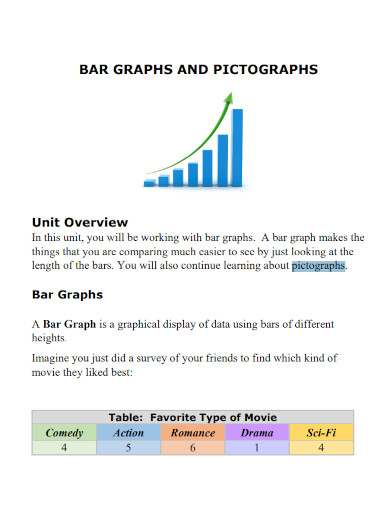virtuallearningacademy.net

## 2. Creating Bar Graph in Numbers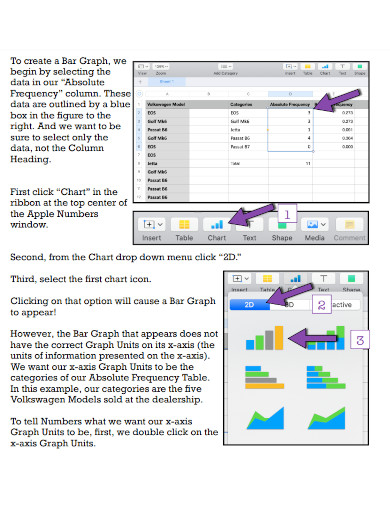online210.psych.wisc.edu

## 3. Sample Data for a Bar Graph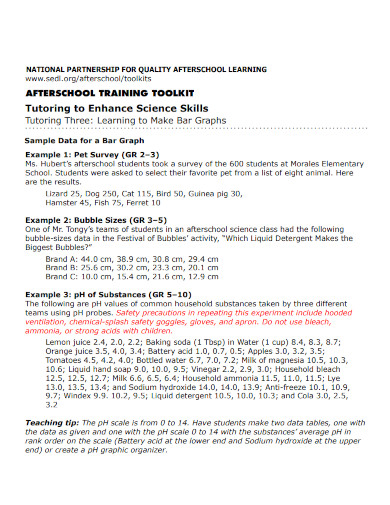sedl.org

## 4. Bar Graphs in SPSS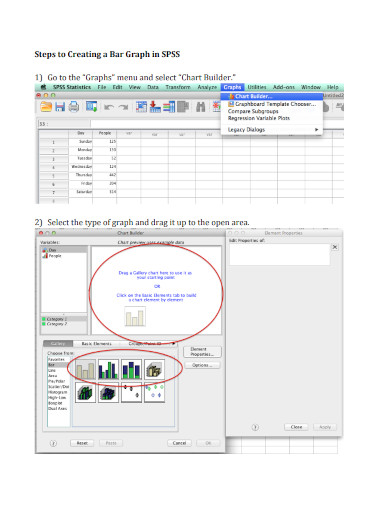westga.edu

## 5. Bar Graphs and Line Graphs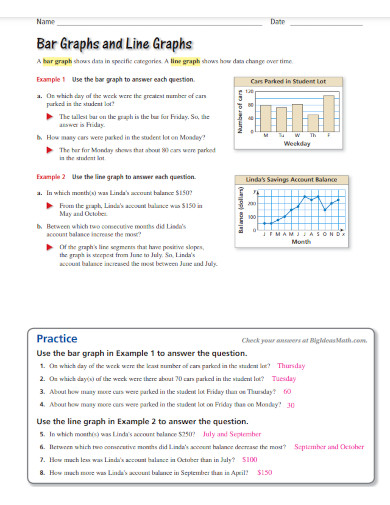static.bigideasmath.com

## 6. Bar Graph Worksheet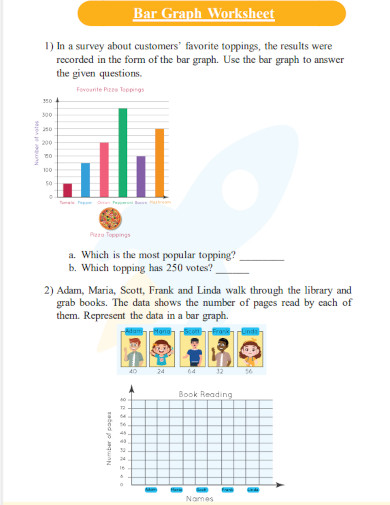d138zd1ktt9iqe.cloudfront.net

## 7. Making  Bar Graph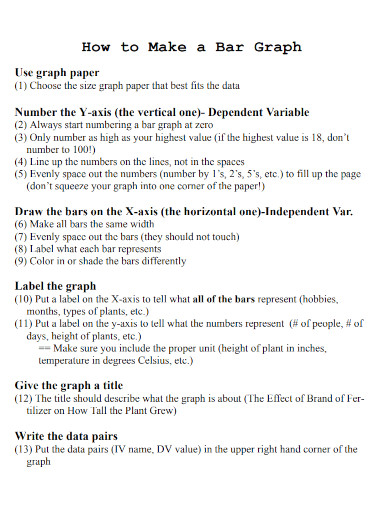thinkscience.org

## 8. Histograms and Bar Graphs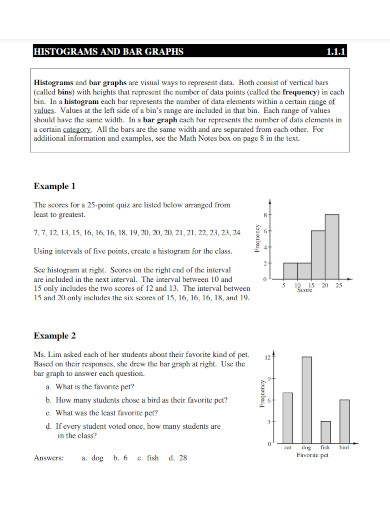mrsmkramer.weebly.com

## 9. Stacked Bar Graphsus.sagepub.com

## 10. Energy Bar Graphs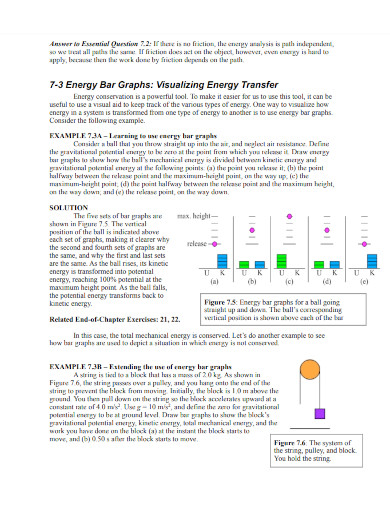webassign.net

## 11. Bar Graphs with Intervals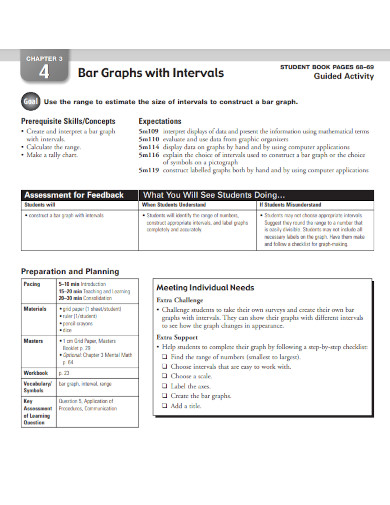mrkerleysclass.yolasite.com

## What Is a Bar Graph

The bar graph is a type of graph that will visually relay or indicate the relationship between the category and a specific numerical value. This visual representation will allow people to efficiently understand the data and create logical connections between the results.

## How to Create a Bar Graph

Bar graphs can easily relay all the numerical information and data through a visual medium, which means that you will need to know how to properly create a bar graph. If you need a reference, you may use any of the bar graph templates, bar graph examples, and samples on the links above.

### Step 1: Determine the Theme and Tone of the Graph

Begin by determining the theme and tone the bar graph will have. This means that if the bar graph is used in a professional context, then the colors and theme of the bar should be professional. But if the bar graph is used in a less-serious manner then you may creatively create your bar graph.

### Step 2: Obtain the Referred Data

You will need the data you will use to illustrate the bar graphs. Therefore, you will need to obtain any relevant and adjacent data sets that the bar graph will represent.

### Step 3: Label the X and Y-Axis

Two axes compose the entirety of the bar graph which are the X-axis (horizontal) and the Y-axis (vertical). The X-axis should represent the category of the data, while the Y-axis represents the numerical value. Be sure to properly label these axes, as they will affect how the data will be interpreted and analyzed.

### Step 4: Using the Data, Create Bars that Will Represent the Relationship Between the X and Y-Axis

Using the data, you will need to create and illustrate bars that will represent the relationship of both the X and Y axis. Connect these points and create bars that will relay the numerical value of the specific category.

### Step 5: If Needed, Analyze the Data

If the situation requires you to analyze the data on the bar graph, you will need to create a conclusory sentence or paragraph that will succinctly talk about the data presented. You can ideate and conduct brainstorming sessions to properly form your conclusory statement.

## Histogram vs. bar graph; what is the difference between a histogram and a bar graph?

There are many types of illustrated graphs we can use to try and visually represent specific statistics for further analysis. A histogram is a graphical representation of the frequency of the data compared to numerical ranges drawn in bar form. This graph will not have spaces between each bar creating a graph with connected bars. A bar graph, on the other hand, is a graphical representation that tries to outline the relationship between categories and their numerical values with one. There is a gap that goes between each bar, in the effort to separate each category. The main difference between a histogram and a bar graph is the values they represent, compare, juxtapose, and contrast with one another.

## Why are bar graphs very important in statistical analysis?

Bar graphs allow the person to visually represent statistical data and information in a visually balanced way with specific colors and identifying marks. There are many different ways to analyze data, but all of these methods require the person to manually compare the numerical values of each category. The bar graph creates a visual reference that makes the statistical analysis easier and more efficient. Not only will the bar graph pool all the data in one whole succinct visual aid, but this will also allow analysts to easily generate insights that may have been missed or overlooked. Therefore bar graphs are important as they will make the analysis less time-consuming and holistic.

## Can you use bar graphs in quantitative and qualitative research?

The scientific method requires researchers to present their discoveries and findings holistically. This means that researchers should be able to provide their raw findings in various methods and ways (see surveys and survey questions). Both quantitative and qualitative research methods can create data and codes, which can be presented in various formats and ways. One can use bar graphs to create a visual representation of the data the researchers have collected. Therefore, you can use bar graphs as a visual representation of the data you have gathered.

The bar graph is a type of graph that is characterized by the presence of bars denoting a specific value in a given category of data. The main objective and goal of the bar graph are to provide a visual representation of the numerical data as a way to make analysis more efficient and effective. Therefore if one wants to easily navigate and analyze data, then one will need to know how to properly create bar graphs.